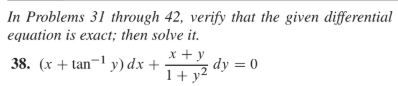# In Problems 31 through 42, verify that the given differential equation is exact; then solve it. 38. (x + tan¬1 y) dx + x +y 1+ y2 dy = 0

Questionhelp_outlineImage TranscriptioncloseIn Problems 31 through 42, verify that the given differential equation is exact; then solve it. 38. (x + tan¬1 y) dx + x +y 1+ y2 dy = 0 fullscreen

### Want to see this answer and more?

Experts are waiting 24/7 to provide step-by-step solutions in as fast as 30 minutes!*

*Response times vary by subject and question complexity. Median response time is 34 minutes and may be longer for new subjects.
Tagged in
MathCalculus

### Differential Equations